Courses

# Additional Questions Solution- Triangles Class 10 Notes | EduRev

## Class 10 : Additional Questions Solution- Triangles Class 10 Notes | EduRev

The document Additional Questions Solution- Triangles Class 10 Notes | EduRev is a part of the Class 10 Course Mathematics (Maths) Class 10.
All you need of Class 10 at this link: Class 10

Q1.In the given figure, DE y BC such that AC = 9 cm, AB = 7.2 cm and AD = 1.8 cm. Find AE.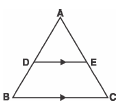Sol. Œ In Δ ABC DE y BC
∴ Using the Basic Proportionality Theorem, we have: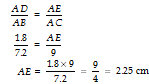Thus, the required value of AE = 2.25 cm.

Q2. In the figure, DE y BC such that AD = 4.8 cm, AE = 6.4 cm and EC = 9.6 cm, find DB.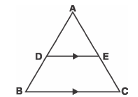Sol. Since DE y BC
∴In Δ ABC, we have
=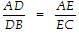[using the Basic Prop. Theorem]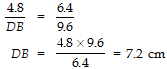⇒ DB = = 7.2 cm
Thus, the required value of DB = 7.2 cm.

Q3. In the given figure, DE y BC such that
AD = (7x - 4) cm, AE = (5x - 2) cm. If EC = 3x and DB = (3x + 4) cm, then find the value of x.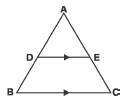Sol. Since, in Δ ABC, DE y BC.
∴ Using Basic Proportionality Theorem, we have
=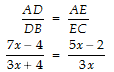⇒ 3x (7x - 4) = (5x - 2) (3x + 4)
⇒ 21x2 - 12x = 15x2 + 20x - 6x - 8
⇒ 21x2 - 15x2 - 12x - 20x + 6x = - 8
⇒ 6x2 - 26x + 8 = 0
⇒ 3x2 - 13x + 4 = 0
⇒ 3x2 - 12x - x + 4 = 0
⇒ 3x (x - 4) - 1 (x - 4) = 0
⇒ (3x - 1) (x - 4) = 0
⇒ x = or 4.

Q4. In the given figure, DE y BC such that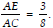. If AB = 4.8 cm then find AD.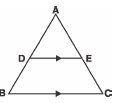Sol. Since DE y BC
∴ Using Basic Proportionality Theorem in D ABC, we have
=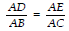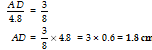Q5.In the given figure, if DE y BC and , then find AC such that AE = 2.1 cm.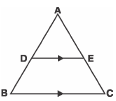Sol. Œ DE y BC
∴In Δ ABC, using the Basic Proportionality Theorem, we have: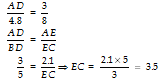Now, AC = AE + EC = (2.1 + 3.5) cm
= 5.6 cm.

Q6. In the figure, D ABC ~ D DEF and AB/DE=3 . If BC = 4 cm then find EF.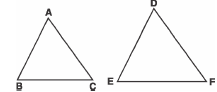Sol. Œ Δ ABC ~ Δ DEF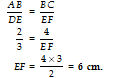.

Q7. The areas of two similar triangles ABC and DEF are 81 cm2 and 100 cm2 respectively.
If EF = 5 cm, then find BC.
Sol.
Œ Δ ABC ~ Δ DEF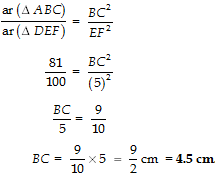Q8. The area of D PQR = 64 cm2. Find the area of D LMN, if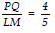and D PQR ~ D LMN.
Sol.
Œ Δ PQR ~ Δ LMN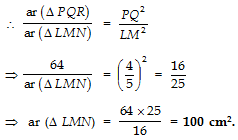Q9. In the figure, <B = 90. Find AC.
Sol.
Œ< B = 90°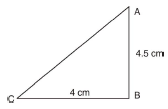∴Δ ABC is a right angled triangle.
∴Using Pythagoras theorem, we have:
AC2 = AB2 + BC2
= (4.5)2 + 42
= 20.25 + 16
= 36.25
⇒ AC = = 6.02 cm (approx.)

Q10. In the figure, if <A = 30° then find the measure of <C.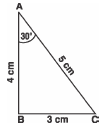Sol. In Δ ABC, we have:
52 = 32 + 42
∴Using the converse of Pythagoras Theorem, we have
Δ ABC is right angled at B; i.e., <B = 90°
Now using the angle-sum-property of a triangle, we get
90° + 30° + <C = 180°
⇒ <C = 180° - 90° - 30° = 60°.

Q11. In the figure, ST y QR, PS = 2 cm and QS = 3 cm. What is the ratio of the area of D PQR to the area of D PST.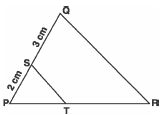Sol. In Δ PQR and Δ PST
Œ <P = <P [Common]
And <PQR = <PST [Œ ST y QR]
∴ ΔPQR ~ Δ PST
[By AA similarity rule]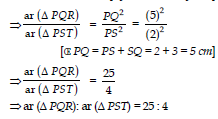Q12. In the figure, DE y AB, find the length of AC.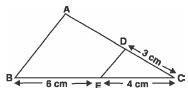Sol. In Δ ABC, we have DE y AB
∴Using the Basic Proportionality Theorem, we have:
=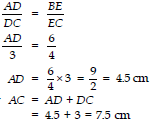Now AC = AD + DC
= 4.5 + 3 = 7.5 cm
Thus, AC = 7.5 cm.

Q13. In < LMN, <L = 50° and <N = 60°. If Δ LMN ~ Δ PQR, then find <Q.
Sol.
By the angle-sum property,
<L + <M + <N = 180°
⇒ 50° + <M + 60° = 180°
⇒ <M = 180° - 60° - 50°
= 70°.
Since, Δ LMN ~ Δ PQR
∴<L = <P
<M = <Q
<N = <R
Now, <Q = <M = 70°
⇒ <Q = 70°.

Q14. In the figure, <M = <N = 46°. Express x in terms of a, b and c where a, b, and c are lengths of LM, MN and NK respectively.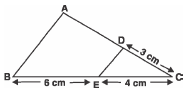Sol. In Δ KML and Δ KNP
<M = <N = 46° [Given]
<MKL = <NKP [Common]
⇒ Δ KML ~ Δ KNP
[AA similarity rule]
∴Their corresponding sides are proportional.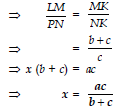Q15. If the areas of two similar triangles are in the ratio 25 : 64, write the ratio of their corresponding sides.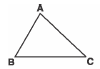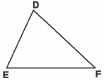Sol. Here, Δ ABC ~ Δ DEF
Since the ratio of the areas of two similar triangles is equal to the ratio of the squares of their corresponding sides,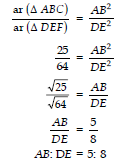Q16. In a Δ ABC, DE y BC. If DE = BC and area of Δ ABC = 81 cm2, find the area of Δ ADE.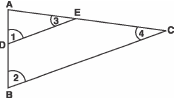Sol. Since DE y BC
∴<1 = <2 [Corresponding angle]
Also <3 = <4 [Corresponding angles]
⇒ Δ DAE ~ Δ BAC [By AA criterion]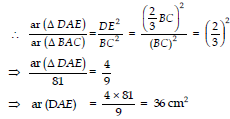Thus, ar D ADE = 36 cm2.

Q17. In the figure, P and Q are points on the sides AB and AC respectively of D ABC such that AP = 3.5 cm, PB = 7 cm, AQ = 3 cm and
QC = 6 cm. If PQ = 4.5 cm, find BC.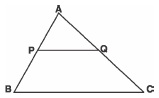Sol. We have: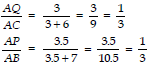In Δ AQP and Δ ACD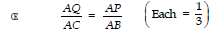⇒ Δ AQP ~ Δ ACB
[SAS similarity rule]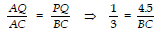[Œ In similar Ds, ratio of
corresponding sides are equal]
⇒ BC = 4.5 × 3 = 13.5 cm.

Q18. In the figure, PQ = 24 cm, QR = 26 cm, <PQR = 90°, PA = 6 cm and AR = 8 cm. Find <QPR.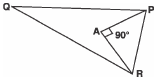Sol.  In right Δ PAR,
By the Pythagoras theorem,
PR2 = PA2 + AR2
= 62 + 82 = 36 + 64 = 100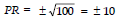But length cannot be negative
∴ PR = 10 cm
Now,
PR2 + PQ2 = 102 + 242
= 100 + 576 = 676
= 262 = QR2
⇒ PR2 + PQ2 = QR2
⇒ <QPR = 90°

Q19. D, E and F are the mid-points of the sides BC, AC and AB respectively of D ABC. Find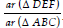.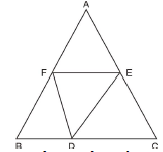Sol. Œ E and F are the mid points of CA
and AB [Given]
∴ By mid point theorem,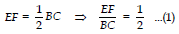...(1)
Similarly,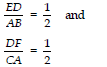...(2)
=                             ...(3)
From (1), (2) and (3), we get
=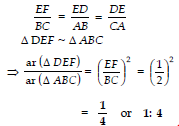Q20. In the figure, PQ y BC and AP : BP = 1 : 2. Find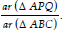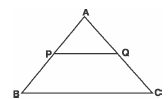Sol. It is given that
AP : PB = 1 : 2
Let AP = k ⇒ PB = 2k
∴ AB = AP + PB = k + 2k = 3k
Œ PQ y BC
∴ <APQ = <ABC and
<AQP = <ACB [Corresponding Angles]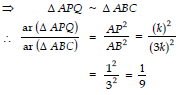Q21. In the figure, DE y BC and . If AC = 4.8 cm, find the length of AE.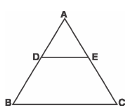Sol. Let AE = x
∴EC = AC - AE
= (4.8 - x) cm
Œ DE y BC [Given]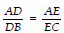[By the Basic Proportionality Theorem]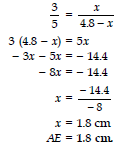Q22. In Δ ABC (shown in the figure), DE y BC. If BC = 8 cm, DE = 6 cm and area of Δ ADE = 45 cm2, then what is the area of Δ ABC?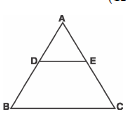Sol. Œ DE y BC
∴In Δ ADE and Δ ABC,
<D = <B and <E = <C
[Corresponding angles]
∴ Δ ADE ~ Δ ABC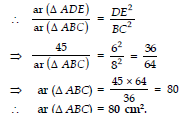Q23. In the figure, DE y BC and AD = 1 cm, BD = 2 cm. What is the ratio of the areas of Δ ABC to the area of Δ ADE?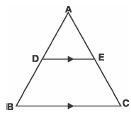Sol. In Δ ABC and Δ ADE
<A = <A [Common]
[Corresponding angles]
∴Using AA similarity, we have: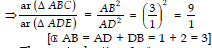⇒ The required ratio = 9 : 1.

Q24. In the figure, AC y BD. Is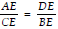?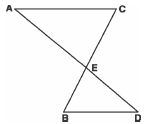Sol. In Δ ACE and Δ DBE,
<A = <D
[Alternate Interior Angles]
<C = <B
∴Using AA similarity
Δ ACE ~ Δ DBE
∴Their corresponding sides are proportional.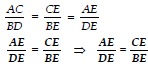Offer running on EduRev: Apply code STAYHOME200 to get INR 200 off on our premium plan EduRev Infinity!

,

,

,

,

,

,

,

,

,

,

,

,

,

,

,

,

,

,

,

,

,

;